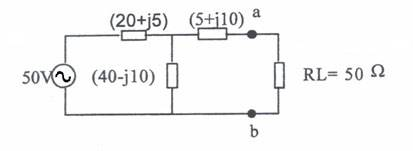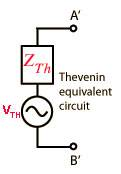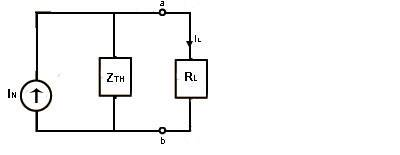# Use Thevenin’s and Norton’s theorems to find the current in RL for the

## Homework Statement

Use Thevenin’s and Norton’s theorems to find the current in RL for the circuit## The Attempt at a Solution

Thevenin equivalentI have calculated VTH and ZTH
VTH=33.793-j5.517
ZTH=19.068+j11.172
Then I converted them in polar forms
Thefore I calculated the current through the load resistor I=VTH/ZTH+RL
I=0.489<-18.401

## Homework Statement

My problem is Norton equivalent.Can it be represented like this:and then IN=VTH/ZTH,once I find IN can easily calculate IL=IN(ZTH/ZTH+RL)

Last edited by a moderator:

gneill
Mentor

## Homework Statement

Use Thevenin’s and Norton’s theorems to find the current in RL for the circuit## The Attempt at a Solution

Thevenin equivalentI have calculated VTH and ZTH
VTH=33.793-j5.517
ZTH=19.068+j11.172
Those values look fine.

Then I converted them in polar forms
Thefore I calculated the current through the load resistor I=VTH/ZTH+RL
I=0.489<-18.401
The current magnitude looks good. Maybe a typo in the second decimal place of the angle?

## Homework Statement

My problem is Norton equivalent.Can it be represented like this:and then IN=VTH/ZTH,once I find IN can easily calculate IL=IN(ZTH/ZTH+RL)
Yes, that's correct.

•1 person